Math resources Number and quantity

Multiplication and division

# Multiplication and division

Here you will learn about multiplication and division, including strategies on how to multiply and divide various types of rational numbers.

Students first learn about multiplication and division in the 3 rd grade and 4th grade with their work with operations and algebraic thinking, as well as number and operations base ten and fractions.

## What is multiplication and division?

Multiplication and division are two of the four basic operations. Multiplication is finding the product of two or more numbers, and division is finding the quotient of two numbers.

Multiplication is basically the repeated addition of equal groups.

For example, 4 equal groups of 3 :

In a multiplication equation, the answer to multiplying one number by another is called the product. The multiplicand is the quantity to be multiplied by the multiplier, which will give you a product.

The product will be 0 if either the multiplicand or multiplier is 0 .

Arrays are visual models that represent multiplication.

For example, this array shows 3 rows of 6 which is the same as 3 \times 6 .

3 \times 6=18

Step by step guide: Understanding Multiplication

Multiplication is commutative. The order in which the calculation is performed does not matter.

For example,

3\times{4}=4\times{3}=12

### Multiplying multi-digit numbers

To multiply multi-digit numbers, you can use the algorithm or the area model.

The area model is a rectangular model where the product represents finding the area of the rectangle.

For example, multiply 42 \times 62 using an area model.

2400+120+80+4=2604

42 \times 62=2604

Step-by-step guide: Multiplying multi-digit numbers

### Multiplicative comparisons

You can use multiplication to make comparisons between quantities. Multiplicative comparisons compare two quantities by showing that one quantity is how many times larger or smaller than another quantity.

For example,

Mike has 3 lollipops. Michelle has 4 times as many lollipops as Mike. How many lollipops does Michelle have?

Michelle has 4\times 3=12 lollipops.

Jillian has 24 inches of hair ribbon. Suzanne has half that amount. How long is Suzanne’s hair ribbon?

Suzanne’s ribbon is \cfrac{1}{2} \times 24=12 \text { inches }

Step by step guide: Multiplicative comparisons

### Multiplying rational numbers

You can multiply rational numbers. Rational numbers include multi-digit numbers, integers, fractions, and decimals. When multiplying positive and negative numbers, the following rules apply:

For example, (-3) \times(-5)=15

Step by step guide: Multiplying and dividing integers

### Division

Division shares or breaks a number into equal sized groups.

For example, the number 12 can be divided into 4 equal groups of 3 .

In a division equation, the answer you get when you divide one number by another is called the quotient.

The word quotient comes from Latin and means ‘how many times.’ When dividing, you are finding out ‘how many times’ a number goes into another number.

For example,

The quotient will only be 0 if the dividend is 0 but the divisor is not.

For example,

0 \div 8=0

8 \div 0=\text {Does not exist }

Step by step guide: Understanding division

Unlike multiplication, division is not commutative. If the order of the numbers within the calculation changes, the result will change.

12 \div 4 4 \div 12

To solve division problems with larger numbers, you can use long division.

For example, 452.1 \div 3

Step-by-step guide: Long division

Step by step guide: Dividing multi-digit numbers

Division can also be done with positive and negative integers, fractions, and decimals. When dividing positive and negative numbers, the following rules apply:

For example, (-20) \div (-5)=4

Step by step guide: Multiplying and dividing integers

Step by step guide: Multiplying and dividing rational numbers

### What is multiplication and division?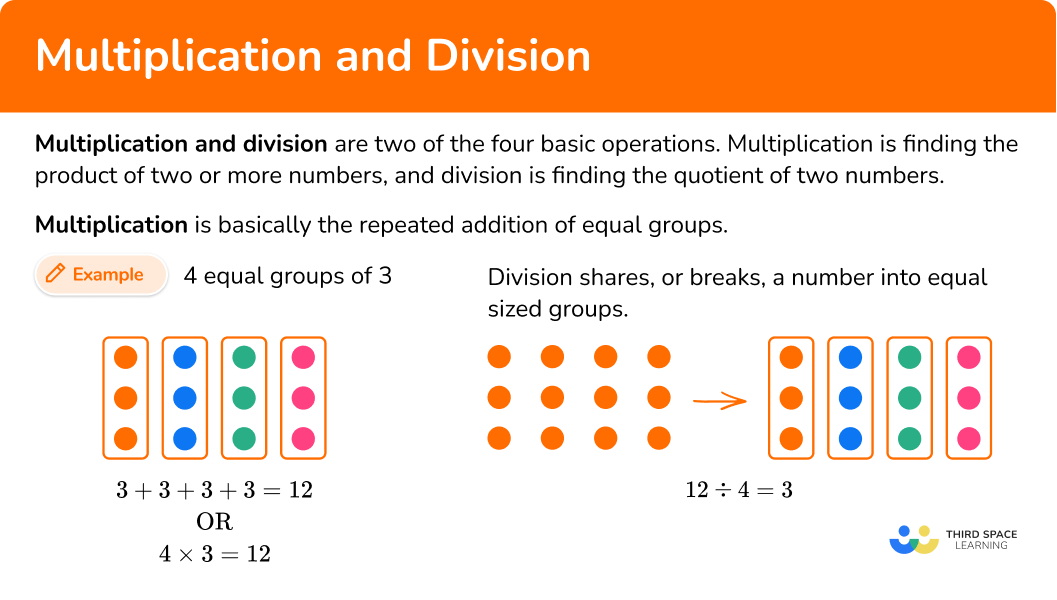## Common Core State Standards

How does this relate to 4 th – 7 th grade math?

• Grade 3: Operations and Algebraic Thinking ( 3.OA.A.1)
Interpret products of whole numbers, for example, interpret 5 × 7 as the total number of objects in 5 groups of 7 objects each.

• Grade 3: Operations and Algebraic Thinking (3.OA.A.2)
Interpret whole-number quotients of whole numbers, for example, interpret 56 \div 8 as the number of objects in each share when 56 objects are partitioned equally into 8 shares, or as a number of shares when 56 objects are partitioned into equal shares of 8 objects each.

• Grade 3: Operations and Algebraic Thinking (3.OA.C.7)
Fluently multiply and divide within 100 , using strategies such as the relationship between multiplication and division.

• Grade 4: Operations and Algebraic Thinking (4.OA.2)
Multiply or divide to solve word problems involving multiplicative comparison, for example, by using drawings and equations with a symbol for the unknown number to represent the problem, distinguishing multiplicative comparison from additive comparison.

• Grade 4: Number and Operations – Fractions (4.NF.B.4)
Apply and extend previous understandings of multiplication to multiply a fraction by a whole number.

• Grade 5: Number and Operations Base Ten (5.NBT.B.5)
Fluently multiply multi-digit whole numbers using the standard algorithm.

• Grade 5: Number and Operations – Fractions (5.NF.B.7)
Apply and extend previous understandings of division to divide unit fractions by whole numbers and whole numbers by unit fractions.

• Grade 6: Number System (6.NS.C.6)
Understand a rational number as a point on the number line. Extend number line diagrams and coordinate axes familiar from previous grades to represent points on the line and in the plane with negative number coordinates.

• Grade 7: Number System (7.NS.A.2)
Apply and extend previous understandings of multiplication and division and of fractions to multiply and divide rational numbers.

## How to do multiplication and division

There are several strategies to multiply and divide numbers. For more specific step-by-step guides, check out the individual pages linked in the “What is multiplication and division?” section above or read through the examples below.

In order to multiply using a visual model:

1. Draw the array.
2. Count the objects in each row.
3. Find the total.

In order to divide using a visual model:

1. Draw the array.
2. Count the objects in each group.

In order to multiply and divide multi-digit numbers:

1. Perform the multiplication or division algorithm.

In order to find multiplicative comparisons:

1. Draw a model.
2. Write an equation.
3. Solve the equation and label the answer.

## Multiplication and division examples

### Example 1: multiply using a model

Use a visual model to multiply 5 \times 3.

1. Draw the array.

5 x 3 is 5 rows of 3.

2Count the objects in each row.

3Find the total.

There are 3 chips in each row, 3+3+3+3+3=15

3 \times 5=15

### Example 2: multiply using algorithm

Multiply 99 \times 7.

Using the algorithm,

99 \times 7=693

### Example 3: multiply and divide rational numbers

Multiply 1.23 \times 3.2.

1.23 \times 3.2=3.936

Divide: 9 \div 3

9 \div 3=3

### Example 5: dividing rational numbers

Divide: 15.4 \div 2

15.4 \div 2=7.7

### Example 6: multiplicative comparison

Bobby has 3 baseball cards. Joey has five times as many cards as Bobby. How many cards does Joey have?

3 \times 5= \, ?

3 \times 5=15

Joey has 15 baseball cards.

### Teaching tips for multiplication and division

• Use manipulatives to reinforce the conceptual understanding of multiplication and division.

• Include real world scenarios so that students can connect the mathematical concepts to the world around them.

• Reinforce to students that the concept of multiplication and division is the same regardless if the numbers are whole numbers or rational numbers.

• Using the area model for multiplication and division can be a fun way for students to understand multiplication and division while also reinforcing math facts.

• To practice multiplication facts, consider using digital and non-digital games instead of flashcards. Game playing is a fun way for students to remember the times tables.

### Easy mistakes to make

• Confusing the rules for multiplying and dividing positive and negative numbers
For example, multiplying (-4)\times (-8) and getting a product of -32 instead of 32 .

• Misinterpreting the meaning of key words in word problems resulting in using the incorrect operation
For example, thinking that the word “of” means to divide instead of multiply.

• Confusing place value when using the area model for multiplying multi-digit numbers
For example, when multiplying 23 \times 41 :

### Practice multiplication and division questions

1) Which multiplication expression represents this array?2 \times 56 \times 25 \times 32 \times 6Count the number of objects in each row.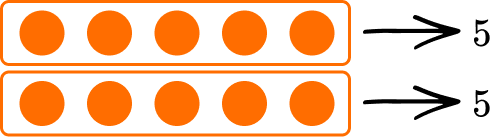There are 5 objects in each row which is 5+5.

5+5 is the same as 2 \times 5.

So, 2 \times 5 is the correct expression.

2) Multiply 104 \times 3.

302321320312Use the algorithm for multiplying multi-digit numbers, regrouping when necessary.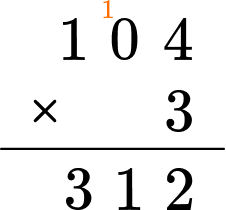104 \times 3=312

3) Multiply 53 \times 32.

1669169616901596You can use the area model to multiply 53 \times 32.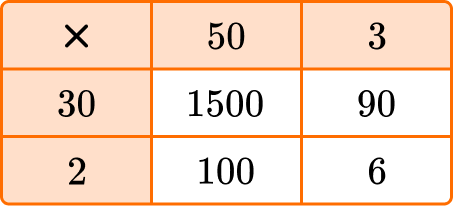53 \times 32=1696

4) Use the array to find the quotient of 16 \div 4 .84166Divide the array into 4 equal groups and then count how many objects are in each group.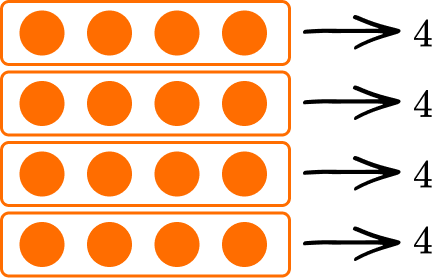16 \div 4 = 4

5) Divide 128 \div 4.

2313232123Divide the numbers using the algorithm for long division.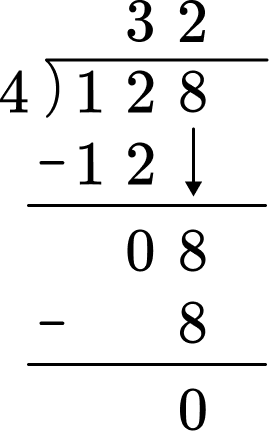128 \div 4= 32

6) Chris has 3 pencils. Pam has four times as many pencils as Chris. How many pencils does Pam have?

11 pencils10 pencils12 pencils7 pencilsDraw a picture.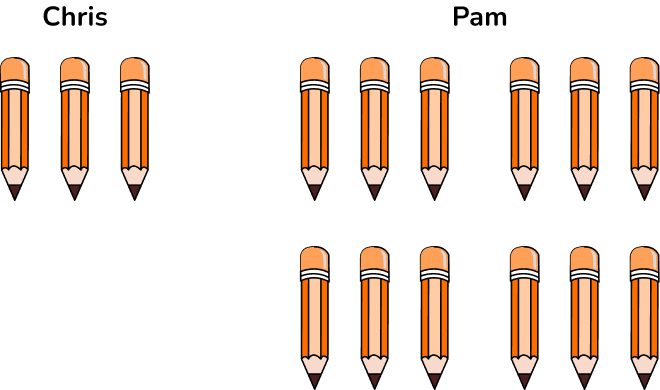4 \times 3=12

Pam has 12 pencils.

## Multiplication and division FAQs

Do the rules for multiplying and dividing positive and negative numbers hold true for all types of numbers?

Yes, the rules for multiplying and dividing positive and negative numbers hold true regardless if the numbers are whole numbers or rational numbers.

Do you need to be really good at multiplication facts and division facts in order to do multiplication problems and division problems?

Knowing your multiplication facts and division facts helps when solving problems.

What is repeated subtraction?

Repeated subtraction is a way for students to begin to develop an understanding of division.

Which method is the best way to do multi-digit multiplication?

There is not one best strategy to use when multiplying multi-digit numbers. Some strategies, can be quicker than others, but not better.

Should you memorize the multiplication tables?

Knowing your multiplication tables helps to answer questions faster than when you do not know them.

What is the commutative property of multiplication?

The commutative property of multiplication is: 5 \times 3=3 \times 5 , the order of the numbers does not matter.

## Still stuck?

At Third Space Learning, we specialize in helping teachers and school leaders to provide personalized math support for more of their students through high-quality, online one-on-one math tutoring delivered by subject experts.

Each week, our tutors support thousands of students who are at risk of not meeting their grade-level expectations, and help accelerate their progress and boost their confidence.